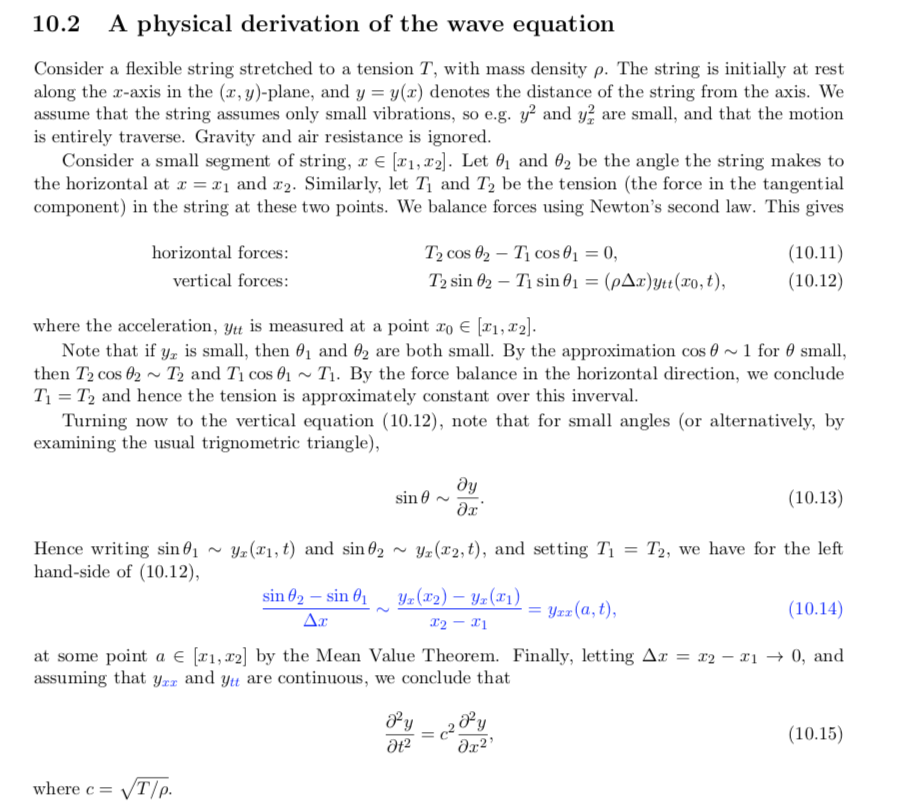# Errata for the 2019-20' MA20223 materials

### Typed notes

• The Corollary 8.4 on convex sets should specify that “if every straight line (that includes a point on the interior of $\Omega$) intersects $\partial\Omega$ at two points at most”.
• There were some typos in the derivation of the wave equation in Chap. 10. It should instead look like this:• Chap. 12 and the Definition 12.8 of the even extension on p.56 should read $x\in[0, L]$ instead of $x\in[0, \pi]$
• Example 15.4 should read $0 < x < \pi$ instead of $0 < x < 2$. (Courtesy DH)
• Eqn (16.7) should have $\lambda^2$ instead of $\lambda$ on the equation for $G$. (Courtesy DH)
• Theorem 18.3 should read the initial conditions of $u(x, 0) = f(x)$ and $u_t(x, 0) = g(x)$. (Courtesy RA)

### Problem sets

• PS5: Q1 should define the thermal conductivity as k not kappa.

### Solutions

• PS3: Bottom of the first page $\partial \phi$ should be $\nabla \phi$
• PS3: Index notation needs to be removed from the main solutions in 19-20' delivery since the topic has been moved to the Appendix. Students are still free to use the technique if they learn it (as noted in lectures).
• PS4 Q4 $dx$ and $dy$ transposed
• PS6 Q1. The function $\sin(x)\exp(-\cos(x^2))$ is indeed not periodic but not for the reasons stated in the solutions. The point here is that $\cos(x^2)$ is not a periodic function. You can verify this either by checking whether it's possible that $(x+L)^2 = x^2 + n\pi$ independent of $x$, or simply by plotting the $\cos($x^2)$and observing its behaviour, particularly near the origin. • PS9 Q2: There is a missing factor of$p$on the bottom here. See Lecture 29. • PS9 Q4: Looks like axes were doubled here. (Courtesy RA, HC) • PS10 Q2: Looks like there are a few$\kappa$s missing on the right hand side (I count three); Q3 In the redefinition of the energy (above “So now you are back to…”) there is a extra d/dt that should not be there. ### Lectures • Correction to lectures 16 when I wrote “Is$\exp(\cos x)$periodic? (No)”. Oops. It is periodic. Note that$\exp(f(x+L)) = \exp(f(x))$. A similar gaff appears in the solutions of PS6. ### Past exams • 2017-18 exam Q4d. The index should start from$n = 1$once the$2n+1\$ shift is done.